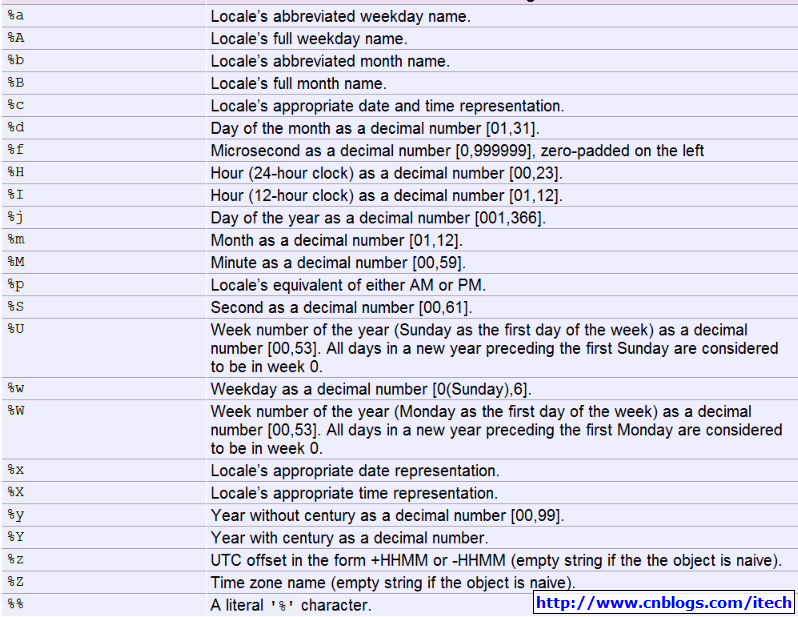# python类库31[时间和日期]

print("-----------------------------------")
#
class datetime.timedelta(days=0, seconds=0, microseconds=0, milliseconds=0, minutes=0, hours=0, weeks=0)
oneyear = datetime.timedelta(days=365)
fivehours
= datetime.timedelta(hours =2)
tenminutes
= datetime.timedelta(minutes = 10)
all
= oneyear + fivehours + tenminutes
print(all)
#365 days, 2:10:00

print("-----------------------------------")
#
datetime.date(year, month, day)
today = datetime.date.today()
print(today)
#2011-01-15
print(today.year)
#2011
print(today.month)
#1
print(today.day)
#15
print(today.weekday())
#5 # Monday is 0 and Sunday is 6
print(today.isoweekday())
#6 #  Monday is 1 and Sunday is 7
print(today.toordinal())
#734152 #the days from 0001.01.01
tomorrow = today.fromordinal(today.toordinal() + 1)
print(tomorrow)
#2011-01-16
print(today.isoformat())
#2011-01-15
print(today.timetuple())
#2011
print(today.timetuple())
#1
print(today.timetuple())
#15
aftertenyears = today.replace(year = 2021)
print(aftertenyears)
#2021-01-15

date1
= datetime.date(2010,10,10)
date2
= datetime.date(2010,9,20)
diff
= date1 - date2 # Diff is datetime.timedelta type
print(diff)
#20 days, 0:00:00
if date1 > date2 :

print("date1 > date2")
#date1 > date2

print("-----------------------------------")
#
datetime.time(hour=0, minute=0, second=0, microsecond=0, tzinfo=None)
time1 = datetime.time(13,30,20)
print(time1)
#13:30:20
print(time1.hour)
#13
print(time1.minute)
#30
print(time1.second)
#20
print(time1.microsecond)
#0
print(time1.isoformat())
#13:30:20
time2 = time1.replace(second = 30)
print(time2)
#13:30:30

print("-----------------------------------")
#class datetime.datetime(year, month, day, hour=0, minute=0, second=0, microsecond=0, tzinfo=None)
print(datetime.datetime.today())
#2011-01-15 14:03:29.495000
print(datetime.datetime.utcnow())
#2011-01-15 06:03:29.495000

#2011-01-15 14:03:29.495000
cdatetime = datetime.datetime.now()
print(cdatetime)
#2011-01-15 14:03:29.495000
print(cdatetime.year)
#2011
print(cdatetime.month)
#1
print(cdatetime.day)
#15
print(cdatetime.hour)
#14
print(cdatetime.minute)
#03
print(cdatetime.second)
#29
print(cdatetime.microsecond)
#495000
print(cdatetime.date())
#2011-01-15
print(cdatetime.time())
#14:03:29.495000
print(cdatetime.weekday())
#5 Monday is 0 and Sunday is 6
print(cdatetime.isoweekday())
#6 Monday is 1 and Sunday is 7
print(cdatetime.isoformat())
#2011-01-15T14:03:29.495000
print(cdatetime.isocalendar())
#(2011, 2, 6)

dt
= cdatetime.strftime("%d %b %Y %I:%M:%S %p"
print(dt)
#15 Jan 2011 02:03:29 PM

dt2
= datetime.datetime.strptime('17 Feb 2009 04:22:11 PM','%d %b %Y %I:%M:%S %p'
print(dt2)
#2009-02-17 16:22:11

=  (2009317162211)
dt3
= datetime.datetime(*k[0:6])
print(dt3)
#2009-03-17 16:22:11

dt4
= dt3.combine(datetime.date.today(),datetime.time(1,10,30))
print(dt4)
#2011-01-15 01:10:30

dt5
= dt3.replace(year = 2015)
print(dt5)
#2015-03-17 16:22:11

dt6
= dt3.fromordinal(dt3.toordinal() + 365)
print(dt6)
#2010-03-17 00:00:00

cdatetime
= datetime.datetime.now()
print(cdatetime)
#2011-01-15 14:03:29.518000
fivedaysago = cdatetime -datetime.timedelta(days=5)
print(fivedaysago)
#2011-01-15 14:03:29.518000
after5hours = cdatetime + datetime.timedelta(hours = 5)
print(after5hours)
#2011-01-15 14:03:29.518000

dt = datetime.datetime.now()
print(dt.strftime("date: %B %d %Y  %A; time: %H:%M:%S ; other: day-%j week-%W"))
#date: January 15 2011  Saturday; time: 15:01:18 ; other: day-015 week-02
print(dt.strftime("%c"))
#01/15/11 15:01:18
print(dt.strftime("%X %x"))
#15:01:18 01/15/11github：https://github.com/cicdops/cicdopsposted @ 2011-01-15 13:25  iTech  阅读(1287)  评论(0编辑  收藏  举报